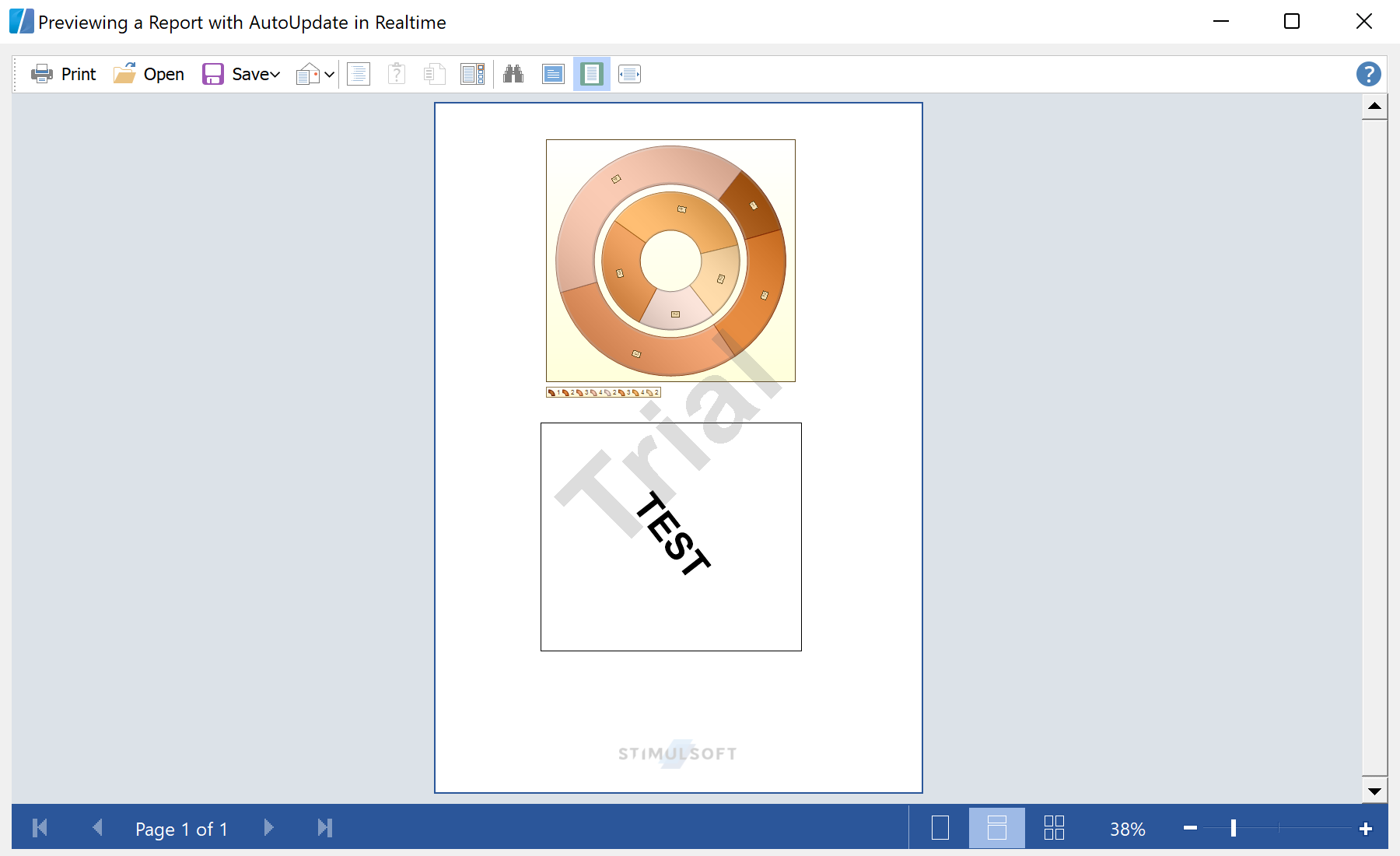This example builds a Realtime Live Report with automatic content update. For example, use a report with some text and a chart with two series. In the Form1() initialization method, find the necessary report components. The report is loaded from the application resources:
private StiText text = null;
private StiChart chart = null;

...

public Form1()
{
//
// Required for Windows Form Designer support
//
InitializeComponent();

stiPreviewControl1.PageViewMode = Stimulsoft.Report.Viewer.StiPageViewMode.Continuous;
stiReport1.Render();
StiComponentsCollection comps = stiReport1.RenderedPages.GetComponents();
text = comps["Text1"] as StiText;
chart = comps["Chart1"] as StiChart;
}

The timer1_Tick timer event change the properties of the selected report components (such as angle), and redraws the report. First, apply the text rotation:
private System.Windows.Forms.Timer timer1;

...

private void timer1_Tick(object sender, System.EventArgs e)
{
if (text == null)return;

// Rotate text
float angle = text.TextOptions.Angle;
angle -= 1f;
if (angle < 0) angle = 359;
text.TextOptions.Angle = angle;

...

Next, since the chart sample has two series, the rotation should be done for each series:
...

// Rotate series 1
angle = ((StiDoughnutSeries)chart.Series).StartAngle;
angle -= 1f;
if (angle < 0) angle = 359;
((StiDoughnutSeries)chart.Series).StartAngle = angle;

// Rotate series 2
angle = ((StiDoughnutSeries)chart.Series).StartAngle;
angle += 1f;
if (angle > 359) angle = 0;
((StiDoughnutSeries)chart.Series).StartAngle = angle;

...

Finally, update the report in realtime:
...

RectangleD rect = stiPreviewControl1.GetComponentRect(text);
stiPreviewControl1.InvalidatePageRect(rect.ToRectangle());

rect = stiPreviewControl1.GetComponentRect(chart);
stiPreviewControl1.InvalidatePageRect(rect.ToRectangle());
//stiPreviewControl1.View.Invalidate();
}

In the screenshot below you can see the result of the sample code: